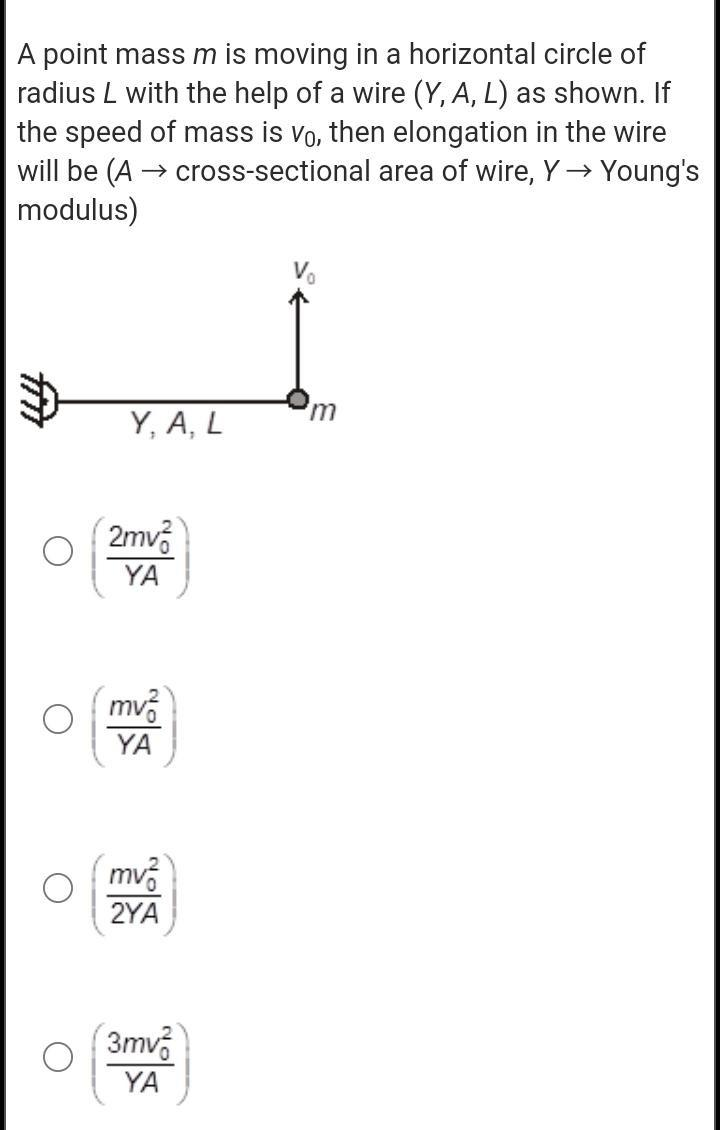Question:

# A point mass m is moving in a horizontal circle of radius L with the help of a wire (Y, A, L) as shown. If the speed of mass isA point mass m is moving in a horizontal circle of radius L with the help of a wire (Y, A, L) as shown. If the speed of mass is vo, then elongation in the wire will be (A → cross-sectional area of wire, Y→ Young's modulus) V m Y, A, L 2mva YA mv. YA O mv. 2YA 3mva YA